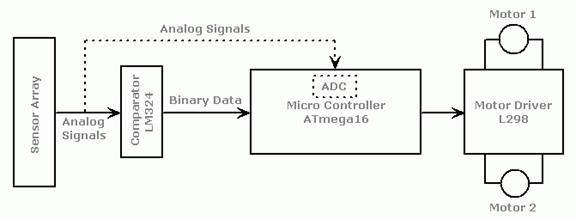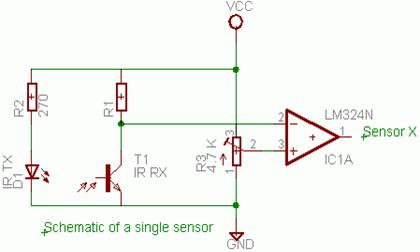# AVR Line Following Robot

Priyank Patil,Block Diagram

The robot uses IR sensors to sense the line, an array of 8 IR LEDs (Tx) and sensors (Rx), facing the ground has been used in this setup. The output of the sensors is an analog signal which depends on the amount of light reflected back, this analog signal is given to the comparator to produce 0s and 1s which are then fed to the µC.

 L4 L3 L2 L1 R1 R2 R3 R4

Left                              Center                               Right

Sensor Array

Starting from the center, the sensors on the left are named L1, L2, L3, L4 and those on the right are named R1, R2, R3, R4.

Let us assume that when a sensor is on the line it reads 0 and when it is off the line it reads 1

The µC decides the next move so as to position the robot such that L1 and R1 both read 0 and the rest read 1.

 L4 L3 L2 L1 R1 R2 R3 R4 1 1 1 0 0 1 1 1

Left                              Center                               Right

Desired State L1=R1=0, and Rest=1

Algorithm:

1.         L= leftmost sensor which reads 0; R= rightmost sensor which reads 0.

If  no sensor on Left (or Right) is 0 then L (or R) equals 0;

For example:

 L4 L3 L2 L1 R1 R2 R3 R4 1 0 0 1 1 1 1 1

Left                              Center                               Right

Here L=3 R=0

 L4 L3 L2 L1 R1 R2 R3 R4 1 1 0 0 0 0 0 0

Left                              Center                               Right

Here L=2 R=4

2.         If all sensors read 1 go to step 3,

else,

If L>R Move Left

If L<R Move Right

If L=R Move Forward

Go to step 4

3.         Move Clockwise if line was last seen on Right

Move Counter Clockwise if line was last seen on Left

Repeat step 3 till line is found.

4.         Go to step 1.Sensor Circuit

For a good voltage swing from the potential divider use

R1= Sqrt (Resistance of sensor when light falls on it * Resistance of sensor when light does not fall on it)

Motor Interface and Control Circuit:The 8 sensors are connected to PORTA.

You need not connect anything to AVCC and AREF, it is required only if ADC is used.

The L298 Motor Driver has 4 inputs to control the motion of the motors and two enable inputs which are used for switching the motors on and off. To control the speed of the motors a PWM waveform with variable duty cycle is applied to the enable pins. Rapidly switching the voltage between Vs and GND gives an effective voltage between Vs and GND whose value depends on the duty cycle of PWM. 100% duty cycle corresponds to voltage equal to Vs, 50 % corresponds to 0.5Vs and so on. The 1N4004 diodes are used to prevent back EMF of the motors from disturbing the remaining circuit.

You may drop your feedback at priyank.patil@gmail.com